Publicité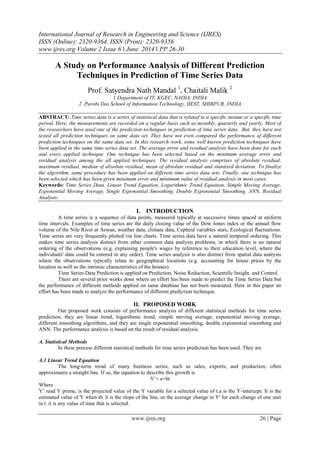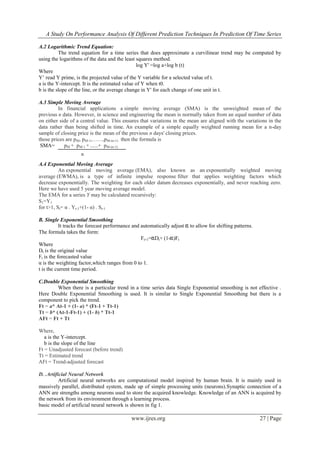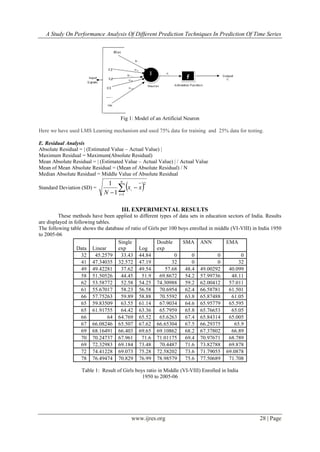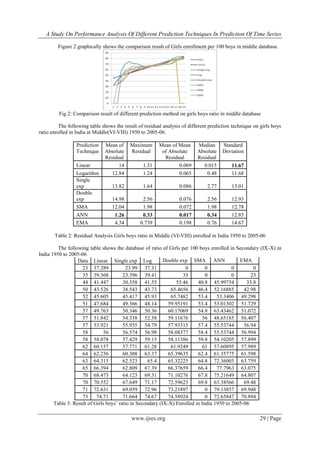Publicité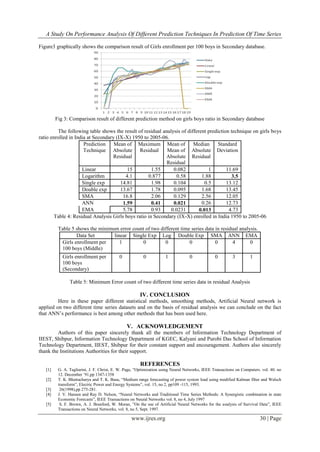Prochain SlideShare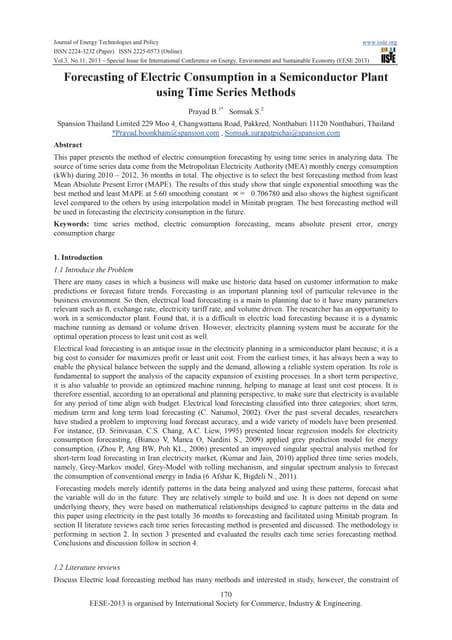Forecasting of electric consumption in a semiconductor plant using time serie...
Chargement dans ... 3
1 sur 5
Publicité

### A Study on Performance Analysis of Different Prediction Techniques in Prediction of Time Series Data

1. International Journal of Research in Engineering and Science (IJRES) ISSN (Online): 2320-9364, ISSN (Print): 2320-9356 www.ijres.org Volume 2 Issue 6 ǁ June. 2014 ǁ PP.26-30 www.ijres.org 26 | Page A Study on Performance Analysis of Different Prediction Techniques in Prediction of Time Series Data Prof. Satyendra Nath Mandal 1 , Chaitali Malik 2 1 Department of IT, KGEC, NADIA, INDIA 2 Purobi Das School of Information Technology, IIEST, SHIBPUR, INDIA ABSTRACT: Time series data is a series of statistical data that is related to a specific instant or a specific time period. Here, the measurements are recorded on a regular basis such as monthly, quarterly and yearly. Most of the researchers have used one of the prediction techniques in prediction of time series data. But, they have not tested all prediction techniques on same data set. They have not even compared the performance of different prediction techniques on the same data set. In this research work, some well known prediction techniques have been applied in the same time series data set. The average error and residual analysis have been done for each and every applied technique. One technique has been selected based on the minimum average error and residual analysis among the all applied techniques. The residual analysis comprises of absolute residual, maximum residual, median of absolute residual, mean of absolute residual and standard deviation. To finalize the algorithm, same procedure has been applied on different time series data sets. Finally, one technique has been selected which has been given minimum error and minimum value of residual analysis in most cases. Keywords: Time Series Data, Linear Trend Equation, Logarithmic Trend Equation, Simple Moving Average, Exponential Moving Average, Single Exponential Smoothing, Double Exponential Smoothing, ANN, Residual Analysis. I. INTRODUCTION A time series is a sequence of data points, measured typically at successive times spaced at uniform time intervals. Examples of time series are the daily closing value of the Dow Jones index or the annual flow volume of the Nile River at Aswan, weather data, climate data, Cepheid variables stars, Ecological fluctuations. Time series are very frequently plotted via line charts. Time series data have a natural temporal ordering. This makes time series analysis distinct from other common data analysis problems, in which there is no natural ordering of the observations (e.g. explaining people's wages by reference to their education level, where the individuals' data could be entered in any order). Time series analysis is also distinct from spatial data analysis where the observations typically relate to geographical locations (e.g. accounting for house prices by the location as well as the intrinsic characteristics of the houses). Time Series Data Prediction is applied on Prediction, Noise Reduction, Scientific Insight, and Control. There are several prior works done where an effort has been made to predict the Time Series Data but the performance of different methods applied on same database has not been measured. Here in this paper an effort has been made to analyze the performance of different prediction technique. II. PROPOSED WORK Our proposed work consists of performance analysis of different statistical methods for time series prediction, they are linear trend, logarithmic trend, simple moving average, exponential moving average, different smoothing algorithms, and they are single exponential smoothing, double exponential smoothing and ANN. The performance analysis is based on the result of residual analysis. A. Statistical Methods In these process different statistical methods for time series prediction has been used. They are A.1 Linear Trend Equation The long-term trend of many business series, such as sales, exports, and production, often approximates a straight line. If so, the equation to describe this growth is Y’= a+bt Where Y’ read Y prime, is the projected value of the Y variable for a selected value of t.a is the Y-intercept. It is the estimated value of Y when t0. b is the slope of the line, or the average change in Y' for each change of one unit in t. it is any value of time that is selected.
2. A Study On Performance Analysis Of Different Prediction Techniques In Prediction Of Time Series www.ijres.org 27 | Page A.2 Logarithmic Trend Equation: The trend equation for a time series that does approximate a curvilinear trend may be computed by using the logarithms of the data and the least squares method. log Y' =log a+log b (t) Where Y’ read Y prime, is the projected value of the Y variable for a selected value of t. a is the Y-intercept. It is the estimated value of Y when t0. b is the slope of the line, or the average change in Y' for each change of one unit in t. A.3 Simple Moving Average In financial applications a simple moving average (SMA) is the unweighted mean of the previous n data. However, in science and engineering the mean is normally taken from an equal number of data on either side of a central value. This ensures that variations in the mean are aligned with the variations in the data rather than being shifted in time. An example of a simple equally weighted running mean for a n-day sample of closing price is the mean of the previous n days' closing prices. those prices are pM, pM-1,……,pM-(n-1) then the formula is SMA= pM + pM-1 + ......+ pM-(n-1) A.4 Exponential Moving Average An exponential moving average (EMA), also known as an exponentially weighted moving average (EWMA), is a type of infinite impulse response filter that applies weighting factors which decrease exponentially. The weighting for each older datum decreases exponentially, and never reaching zero. Here we have used 5 year moving average model. The EMA for a series Y may be calculated recursively: S1=Y1 for t>1, St= α . Yt-1+(1- α) . St-1 B. Single Exponential Smoothing It tracks the forecast performance and automatically adjust to allow for shifting patterns. The formula takes the form: Ft+1= Dt+ (1- )Ft Where Dt is the original value Ft is the forecasted value α is the weighting factor,which ranges from 0 to 1. t is the current time period. C.Double Exponential Smoothing When there is a particular trend in a time series data Single Exponential smoothing is not effective . Here Double Exponential Smoothing is used. It is similar to Single Exponential Smoothing but there is a component to pick the trend. Ft = a* At-1 + (1- a) * (Ft-1 + Tt-1) Tt = b* (At-1-Ft-1) + (1- b) * Tt-1 AFt = Ft + Tt Where, a is the Y-intercept. b is the slope of the line Ft = Unadjusted forecast (before trend) Tt = Estimated trend AFt = Trend-adjusted forecast D. .Artificial Neural Network Artificial neural networks are computational model inspired by human brain. It is mainly used in massively parallel, distributed system, made up of simple processing units (neurons).Synaptic connection of a ANN are strengths among neurons used to store the acquired knowledge. Knowledge of an ANN is acquired by the network from its environment through a learning process. basic model of artificial neural network is shown in fig 1. n
3. A Study On Performance Analysis Of Different Prediction Techniques In Prediction Of Time Series www.ijres.org 28 | Page Fig 1: Model of an Artificial Neuron Here we have used LMS Learning mechanism and used 75% data for training and 25% data for testing. E. Residual Analysis Absolute Residual = | (Estimated Value – Actual Value) | Maximum Residual = Maximum(Absolute Residual) Mean Absolute Residual = | (Estimated Value – Actual Value) | / Actual Value Mean of Mean Absolute Residual = (Mean of Absolute Residual) / N Median Absolute Residual = Middle Value of Absolute Residual Standard Deviation (SD) =     n i xx N i 1 2 1 1 III. EXPERIMENTAL RESULTS These methods have been applied to different types of data sets in education sectors of India. Results are displayed in following tables. The following table shows the database of ratio of Girls per 100 boys enrolled in middle (VI-VIII) in India 1950 to 2005-06 Table 1: Result of Girls boys ratio in Middle (VI-VIII) Enrolled in India 1950 to 2005-06 Data Linear Single exp Log Double exp SMA ANN EMA 32 45.2579 33.43 44.84 0 0 0 0 41 47.34035 32.572 47.19 32 0 0 32 49 49.42281 37.62 49.54 57.68 48.4 49.00292 40.099 58 51.50526 44.45 51.9 69.8672 54.2 57.99736 48.11 62 53.58772 52.58 54.25 74.30988 59.2 62.00412 57.011 61 55.67017 58.23 56.58 70.6954 62.4 66.58781 61.501 66 57.75263 59.89 58.88 70.5592 63.8 65.87488 61.05 65 59.83509 63.55 61.14 67.9034 64.6 65.95779 65.595 65 61.91755 64.42 63.36 65.7959 65.8 65.76653 65.05 66 64 64.769 65.52 65.6263 67.4 65.84314 65.005 67 66.08246 65.507 67.62 66.65304 67.5 66.29375 65.9 69 68.16491 66.403 69.65 69.10862 68.2 67.37802 66.89 70 70.24737 67.961 71.6 71.01175 69.4 70.97671 68.789 69 72.32983 69.184 73.48 70.4487 71.6 73.82788 69.878 72 74.41228 69.073 75.28 72.58202 73.6 71.79055 69.0878 78 76.49474 70.829 76.99 78.98579 75.6 77.50689 71.708
4. A Study On Performance Analysis Of Different Prediction Techniques In Prediction Of Time Series www.ijres.org 29 | Page Figure 2 graphically shows the comparison result of Girls enrollment per 100 boys in middle database. Fig 2: Comparison result of different prediction method on girls boys ratio in middle database The following table shows the result of residual analysis of different prediction technique on girls boys ratio enrolled in India at Middle(VI-VIII) 1950 to 2005-06. Prediction Technique Mean of Absolute Residual Maximum Residual Mean of Mean of Absolute Residual Median Absolute Residual Standard Deviation Linear 14 1.31 0.069 0.015 11.67 Logarithm 12.84 1.24 0.065 0.48 11.68 Single exp 13.82 1.64 0.086 2.77 13.01 Double exp 14.98 2.56 0.076 2.56 12.93 SMA 12.04 1.98 0.072 1.98 12.78 ANN 1.26 0.33 0.017 0.34 12.85 EMA 4.34 0.739 0.198 0.76 14.67 Table 2: Residual Analysis Girls boys ratio in Middle (VI-VIII) enrolled in India 1950 to 2005-06 The following table shows the database of ratio of Girls per 100 boys enrolled in Secondary (IX-X) in India 1950 to 2005-06 Data Linear Single exp Log Double exp SMA ANN EMA 23 37.289 23.99 37.31 0 0 0 0 35 39.368 23.396 39.41 35 0 0 23 44 41.447 30.358 41.55 55.46 40.8 45.99754 33.8 50 43.526 38.543 43.73 65.4656 46.4 52.16885 42.98 52 45.605 45.417 45.93 65.7482 53.4 53.3406 49.298 51 47.684 49.366 48.14 59.95191 53.4 53.01302 51.729 57 49.763 50.346 50.36 60.17069 54.8 63.43462 51.072 57 51.842 54.338 52.58 59.11676 56 48.65185 56.407 57 53.921 55.935 54.79 57.93315 57.4 55.53744 56.94 58 56 56.574 56.98 58.08377 58.4 55.53744 56.994 58 58.078 57.429 59.15 58.11386 59.8 54.10205 57.899 62 60.157 57.771 61.28 61.9249 61 57.60895 57.989 64 62.236 60.308 63.37 65.39635 62.4 61.35775 61.598 63 64.315 62.523 65.4 65.32225 64.8 72.36003 63.759 65 66.394 62.809 67.39 66.37659 66.4 77.7963 63.075 70 68.473 64.123 69.31 71.10276 67.8 75.21649 64.807 70 70.552 67.649 71.17 72.59623 69.8 63.38566 69.48 71 72.631 69.059 72.96 73.21897 0 79.13857 69.948 73 74.71 71.664 74.67 74.58924 0 72.65847 70.894 Table 3: Result of Girls boys’ ratio in Secondary (IX-X) Enrolled in India 1950 to 2005-06
5. A Study On Performance Analysis Of Different Prediction Techniques In Prediction Of Time Series www.ijres.org 30 | Page Figure3 graphically shows the comparison result of Girls enrollment per 100 boys in Secondary database. Fig 3: Comparison result of different prediction method on girls boys ratio in Secondary database The following table shows the result of residual analysis of different prediction technique on girls boys ratio enrolled in India at Secondary (IX-X) 1950 to 2005-06. Prediction Technique Mean of Absolute Residual Maximum Residual Mean of Mean of Absolute Residual Median Absolute Residual Standard Deviation Linear 15 1.55 0.082 1 11.69 Logarithm 4.1 0.877 0.58 1.88 3.5 Single exp 14.81 1.98 0.104 0.5 13.12 Double exp 13.67 1.78 0.095 1.68 13.45 SMA 16.8 2.06 0.129 2.56 12.05 ANN 1.59 0.41 0.021 0.26 12.73 EMA 5.78 0.93 0.0231 0.013 4.73 Table 4: Residual Analysis Girls boys ratio in Secondary (IX-X) enrolled in India 1950 to 2005-06 Table 5 shows the minimum error count of two different time series data in residual analysis. Data Set linear Single Exp Log Double Exp SMA ANN EMA Girls enrollment per 100 boys (Middle) 1 0 0 0 0 4 0 Girls enrollment per 100 boys (Secondary) 0 0 1 0 0 3 1 Table 5: Minimum Error count of two different time series data in residual Analysis IV. CONCLUSION Here in these paper different statistical methods, smoothing methods, Artificial Neural network is applied on two different time series datasets and on the basis of residual analysis we can conclude on the fact that ANN’s performance is best among other methods that has been used here. V. ACKNOWLEDGEMENT Authors of this paper sincerely thank all the members of Information Technology Department of IIEST, Shibpur, Information Technology Department of KGEC, Kalyani and Purobi Das School of Information Technology Department, IIEST, Shibpur for their constant support and encouragement. Authors also sincerely thank the Institutions Authorities for their support. REFERENCES  G. A. Tagliarini, J. F. Christ, E. W. Page, “Optimization using Neural Networks, IEEE Transactions on Computers. vol. 40. no 12. December ’91,pp 1347-1358  T. K. Bhattacharya and T. K. Basu, “Medium range forecasting of power system load using modified Kalman filter and Walsch transform”, Electric Power and Energy Systems”, vol. 15, no 2, pp109 -115, 1993.  26(1998),pp 275-281.  J. V. Hansen and Ray D. Nelson, “Neural Networks and Traditional Time Series Methods: A Synergistic combination in state Economic Forecasts”, IEEE Transactions on Neural Networks vol. 8, no 4, July 1997  S. F. Brown, A. J. Branford, W. Moran, ”On the use of Artificial Neural Networks for the analysis of Survival Data”, IEEE Transactions on Neural Networks, vol. 8, no 5, Sept. 1997.
Publicité